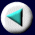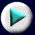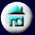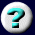12. Heat, Energy, and Chemical        BondsHeat and Chemical Reactions
 The heat given off is 116 kcal for the molar reaction as written; that is, per two moles of hydrogen gas and one mole of oxygen used, and two moles of water vapor formed. If, without thinking, we were to add to this reaction, the reaction H2O(g) ® H2O(l) DH = -10 kcal mole-1 then the result would be not what we want at all: 2H2(g) + O2(g) ® H2O(g) + H2O(l) DH = -126 kcal Although the equation would be true, it would not be particularly useful. One must keep the stoichiometry (the relative numbers of reactant and product molecules) correct for the heats of reaction to be meaningful.
Page 12 of 39